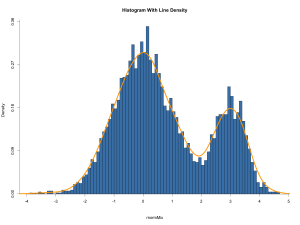# R Graphics Gallery

An  R graphics gallery has been created with simple scripts for long-term reference and to expedite data visualization.

#### Box Plot

In order to access the values behind the chart, simply set the boxplot() argument plot=FALSE.  A statistical summary is generated.

#### HistogramClick to enlarge

The hist() function returns a useful statistical summary if the argument plot=FALSE is used.  The summary defines the breakpoints for the bins and counts per bin.  For example:

The following example shows how bin summary data can be used.  Function pretty() takes a vector argument and divides its range into n equally spaced, ordered values, as shown in the histogram below:

Pie Chart

QQNorm Plot